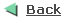Building .NET Controls @ Runtime

Martin Zahn, 27.03.2008

Overview

.NET offers the possibility to create controls in Windows Form at runtime. Often we encounter a situation where we need to load controls dynamically on the form, as well as position and align them properly. Generally we do this when we are not sure about the number of controls to be displayed.

One problem you may encounter happens when you must register a specific event handler to each of the controls. It is not possible to add extra custom parameters to the registered event handler. However, in most cases there is a way to find out which control has fired the event and to react in a proper way within the event handler for the called control.

Example

In the example below we will demonstrate how to build .NET controls at runtime and how a central event handler can be used for all controls. We dynamically create 16 text boxes and attach a KeyPressEventHandler to each of the text boxes.Within the KeyPressEventHandler we verify which of the text box has fired the event with the following code segment.

if (sender is TextBox)
{

TextBox textBox = (TextBox)sender;

int index = Convert.ToInt32(textBox.Tag);
...
}

The variable index holds the text box index, we attached this index when we created the text box.

this.pinTextBox[i].Tag = i;

As an example for the KeyPressEventHandler we move the input focus to the next text box, if the user typed 2 characters.

Here is the full code.

using System;
using System.Collections.Generic;
using System.ComponentModel;
using System.Data;
using System.Drawing;
using System.Text;
using System.Windows.Forms;

namespace DynControl
{

// Building .NET Controls @ Runtime

public class DynControl : Form
{

private TextBox[] pinTextBox = null;

private int xLoc = 0, yLoc = 20, xOffset = 26;

private int numTextBox = 16;

private string strTextBox;

public DynControl()
{

// Create Form
InitializeComponent();

// Create Array of Text Boxes

this.pinTextBox = new TextBox[numTextBox];

// Create each Text Box dynamically

for (int i = 0; i < this.pinTextBox.Length; i++)
{

this.pinTextBox[i] = new TextBox();

this.pinTextBox[i].Location =

new System.Drawing.Point(xLoc += xOffset, yLoc);

this.pinTextBox[i].MaxLength = 2;

this.pinTextBox[i].Name =
"TextBox"
+ i.ToString();

this.pinTextBox[i].Size =

new System.Drawing.Size(25, 20);

this.pinTextBox[i].TabIndex = i + 10;

// Attach the Index of the Text Box
// to the Tag Property, this Index
// is later used in the KeyPress Event Handler

this.pinTextBox[i].Tag = i;

// Asscociate KeyPress Event Handler to each Text Box

this.pinTextBox[i].KeyPress +=

new KeyPressEventHandler(this.SetFocus);

// Add the TextBox to the Form

}
}

// Move Focus to the next TextBox if
// max User-Input is reached

private void SetFocus(object sender, KeyPressEventArgs e)
{

if (sender is TextBox)
{
TextBox textBox = (TextBox)sender;

int index = Convert.ToInt32(textBox.Tag);

this.strTextBox += e.KeyChar;

if (this.strTextBox.Length == 2)
{

if (index < (pinTextBox.Length - 1))
{

this.pinTextBox[index + 1].Focus();
}

this.strTextBox = String.Empty;
}
}
}

// Create Form
private void InitializeComponent()
{

this.SuspendLayout();

this.AutoScaleDimensions =

new System.Drawing.SizeF(6F, 13F);

this.AutoScaleMode =
System.Windows.Forms.
AutoScaleMode.Font;

this.ClientSize = new System.Drawing.Size(464, 64);

this.Name = "DynControl";

this.Text = "Building .NET Controls @ Runtime";

this.ResumeLayout(false);
}
}
}